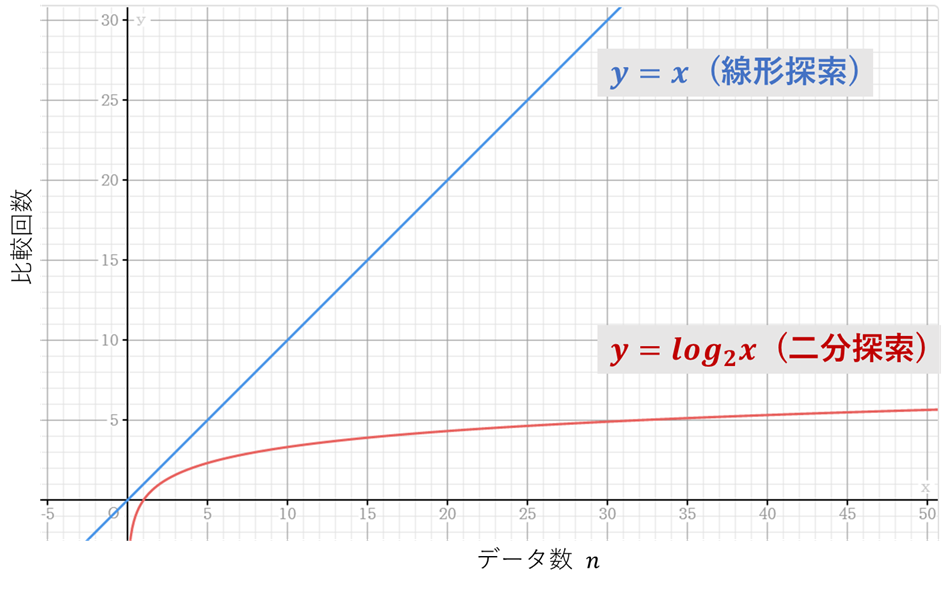# #Algorithm learned in Python

## Introduction

Implement the basic algorithm in Python to deepen your understanding of the algorithm. Binary search is dealt with as the 10th bullet.

## Binary search

When you look at a value, such as the median, you determine whether that value is before or after it. ・ The search range is narrowed by half (divided into two) ⇒ Speed ​​up ※Note ** Data must be arranged regularly, such as in alphabetical order ** In some cases, it may be necessary to sort the data in advance.

## Difference from linear search

The order is as follows. Linear search: \$ O (n) \$ Binary search: \$ O (log_2n) \$ The relationship is shown in a graph for a more intuitive understanding.For example, if there are 1000 or 1 million pieces of data, a linear search requires 1000 or 1 million comparisons, but a binary search requires only 10 or 20 comparisons.

Although there is a restriction that the data must be arranged regularly, it can be seen that the binary search is effective when the amount of data is large. However, when there is little data, there is no big difference in speed, so a linear search that is not related to the data arrangement is often used.

## Implementation

Two implementations are made for binary search. The first is when data that is regularly arranged is given, and the second is that it can be sorted, but it is assumed that it is arranged irregularly and needs to be sorted. The code and its output are shown below.

#### `binary_search.py`

``````
"""
2020/12/22
@Yuya Shimizu

Binary search
"""

def binary_search(data, target):
left = 0                    #Set the left edge of the search range
right = len(data) - 1       #Set the right edge of the search range

while left <= right:
mid =  (left + right) // 2         #Median range to search
if data[mid] == target:
return mid                     #Returns the position if it matches the median
elif data[mid] < target:
left = mid + 1                 #If it is larger than the median, change the left edge of the search range.
else:
right = mid - 1                #If it is smaller than the median, change the right edge of the search range.

return False

if __name__ == '__main__':
data = [10, 20, 30, 40, 50, 60, 70, 80, 90]
target = 5

found = binary_search(data, target)
else:
print(f"{target} is found at data[{found}]")
``````
##### Output (when data is found)
``````50 is found at data
``````
##### Output (when no data is found)
``````5 is not found in the data
``````

#### `binary_search.py`

``````
"""
2020/12/22
@Yuya Shimizu

Binary search
"""

def binary_search(data, target):
data_sorted = sorted(data)  #Sort the data in ascending order(data, reverse=True)Then you can do it in descending order

left = 0                    #Set the left edge of the search range
right = len(data) - 1       #Set the right edge of the search range

while left <= right:
mid =  (left + right) // 2         #Median range to search
if data_sorted[mid] == target:
return mid , data_sorted       #If it matches the median, the data sorted by that position is returned.
elif data_sorted[mid] < target:
left = mid + 1                 #If it is larger than the median, change the left edge of the search range.
else:
right = mid - 1                #If it is smaller than the median, change the right edge of the search range.

return False, -1

if __name__ == '__main__':
data = [10, 50, 60, 20, 30, 40, 90, 70, 80]
target = 50

found, data_sorted = binary_search(data, target)

else:
print(f"data_sorted is the sorted data : \n{data} >>> {data_sorted}\n")
print(f"{target} is found at data_sorted[{found}]")
``````
##### Output (when data is found)
``````data_sorted is the sorted data :
[10, 50, 60, 20, 30, 40, 90, 70, 80] >>> [10, 20, 30, 40, 50, 60, 70, 80, 90]

50 is found at data_sorted
``````
##### Output (when no data is found)
``````5 is not found in the data
``````

I don't know if the second one is necessary, but I tried to make it like this if I made a function that also includes sorting. I think it will work if it is a sortable data list. The search range is reduced by repeatedly replacing the left and right edges with the median.

## Impressions

I learned that there is such a difference from linear search. Although it is natural from the mechanism, I was able to understand it more intuitively by displaying it in a graph.

## References

Introduction to algorithms starting with Python: Standards and computational complexity learned with traditional algorithms Written by Toshikatsu Masui, Shoeisha